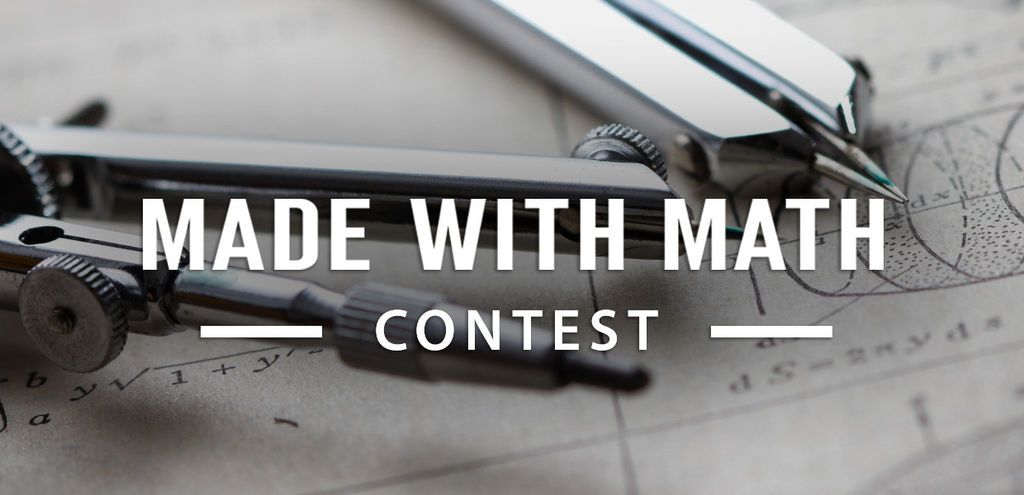# Using Napier's Bones

461

4

John Napier was a late 16th/17th century, Scottish mathematician and inventor, although I consider him to be an Engineer. He was obsessed with simplifying mathematical calculating and his work led to him discovering logarithms. It is ironic that generating logarithmic tables, a method that eliminated the need to multiply, required the multiplication of a lot of very large numbers. To simplify the process he use lattice multiplication and invented his bones to reduce errors and increase the speed of filling out large lattices.

Included with this Instructable is a PDF file to print a set of bones on 11" x 17" paper or tagboard. Also included is a SVG file to make a set with a laser using 12" x 12" plywood or MDF.

The set consists of 30 bones (three sets of 0-9 bones.), an index bone, a square root bone, and an answer grid. A PDF, of easy to understand instructions to multiply, is also included. Make yourself a set and follow along.

### Teacher Notes

Teachers! Did you use this instructable in your classroom?
Add a Teacher Note to share how you incorporated it into your lesson.

## Step 1: Lattice Multiplication

Lattice multiplication uses a lattice to multiply two multi digit numbers. It is the same method used for long multiplication but breaks the problem into smaller steps that are easier to understand and less prone to error.

The best way to show how it works is to do an example. 1423 * 175 = 249,025.

You will need to draw 12 boxes, four wide and three tall. In each box draw a diagonal from the top right to the bottom left. This is your lattice.

Write your four digit value, from left to right, on the top of the lattice. Then write your three digit value, for top to bottom the right side of the of the lattice.

In each of the 12 boxes, write the value of the multiplication of its values on top and its value to the right. Write the 1s digit in the lower right triangle of the box. If there is a 10s digit write it in the top left triangle of the box.

Starting on the right, add the values between the diagonals. Remember to pass the carry to the next diagonal on the left. Reading the answer from the top left you can see it is 2 4 9 0 2 5.

## Step 2: Napier's Bones

The photo shows the 10 different basic bones alone with the index bone. You can see the each bone has the nine multiples of its top value. For example, the "3 Bone" has values 3, 6, 9, 12, 15, 18, 21, 24, and 27. All the single digit multiples of 3. With these ten bones and index, you have all the multiples of two single digits.

Note: These bones were laser cut from 1/8" MDF. If you do not have access to a laser then use the PDF to print your bones on tag board of some other thick paper product.

## Step 3: Lattice Multiplication With the Bones.

Lets fill out the same lattice that was used in Step 1. Just like in Step 1 write the value 1423 across the top, from left to right, and the value 175 on the right, from top down.

Take "1","4", "2", and "3" bones, along with the index bone, and place them as shown in this step's first photo.

Use the alignment grid to shown the Index 1 row. Transfer the bone value to the top four boxes of the lattice. Note they match those calculated in Step 1.

Move the grid to show the Index 7 row and transfer the shown bone values to the center four boxes of the lattice.

Move the grid to show the Index 5 row and transfer the shown bone values to thee remaining bottom four boxes of the lattice.

Note that all the values in the lattice are the same as those you calculated in Step 1.

This is best video I have found on multiplying with Napier's bone can be found here.

## Step 4: Napier's Square Root Bone

To generate a logarithm table all that is needed is the ability to multiply large numbers and take the square root of the result

Basically:

log(1) = 0

log(10) = 1

log (√(a•b) = (log(a) + log(b))

Given two values and their logs another value and its log can be found between them. This means the given the first two values all the other points can be found with enough effort.

The square root bone is shown in the photo above. Instead of trying to explain it operation, I will send you to this video.

He does a far better job explaining it than I ever could.

## Step 5: All in a Box

All

these 30 bones, index bone, square root bone, and answer grid all fit into a 6" x 6" x 1-1/4" mailer that a found on Amazon.

Boxes

There is plenty of room to add up to 30 more bones, if desired.

One last thing. The same guy that did the other two videos also has another on long division with Napier's Bones. It's worth a look.

ps Don't you just love his accent.Participated in the

## Recommendations

95 9.7K
32 8.7K
82 13KLamps Class

9,865 Enrolled